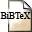# Optimal channel allocation in quasi-linear and quasi-cyclic cellular radio networks

## Abstract

We investigate channel assignment problems for two general classes of cellular radio networks. The blocking probability of a network is chosen as optimization criterion. Two divide-and-conquer algorithms are presented which for both types of networks determine an optimal allocation of one channel. By iteratively applying these algorithms an abitrary number of channels may be allocated efficently. This method yields a blocking probability close to the optimum.

## BibTEX Reference Entry```@inbook{MaMa94,
author = {Rudolf Mathar and J{\"u}rgen Mattfeldt},
title = "Optimal channel allocation in quasi-linear and quasi-cyclic cellular radio networks",
pages = "760-766",
series = "IMACS Symp. Math. Mod.",
editor = "I. Troch, F. Breitenecker",Download bibtex-file